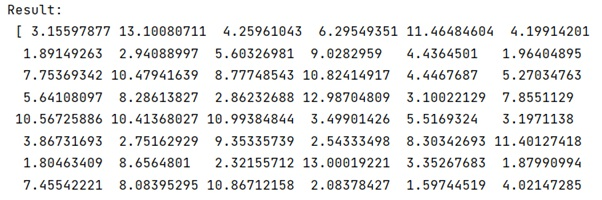# Generate random array of floats between a range

Learn, how to generate random array of floats between a range? By Pranit Sharma Last updated : October 08, 2023

NumPy is an abbreviated form of Numerical Python. It is used for different types of scientific operations in python. Numpy is a vast library in python which is used for almost every kind of scientific or mathematical operation. It is itself an array which is a collection of various methods and functions for processing the arrays.

Floats are reusable code in the programming language that converts values into floating point numbers. It contains all the decimal values or fractional values like 333.2232, 554.23432, 4793.4242.

Here, we need to define a function to generate a random array of floats between a certain range.

## Generating random array of floats between a range

For this purpose, we are going to use numpy.random.uniform() method which can be used to generate a random series of values in a certain range where the range can be defined with the 'low' and 'high' arguments.

Let us understand with the help of an example,

## Python program to generate random array of floats between a range

```# Import numpy
import numpy as np

# Creating a random series of float values
res = np.random.uniform(low=0.5, high=13.3, size=(50,))

# Display series of float values
print("Result:\n",res)
```

### Output

The output of the above program is: